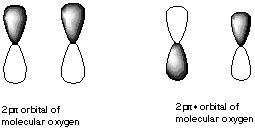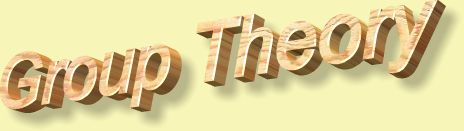1. Identify the point group of each of the following pictures. Which are chiral? Which have dipole moments? (Your're going to have to imagine that these drawings are more perfect than they here appear.)

A. five-pointed star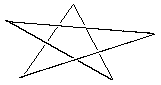B. Star of David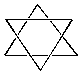C. baseball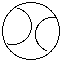D. pencil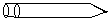2.* (1997 F 12) The compound tris (ethylenediamine) cobalt (III), Co (H2NCH2CH2NH2) 33-, has the structure pictured below: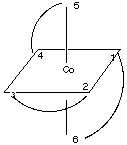where the curved lines represent the three bidentate en ligands, each one of which is attached to the Co atom by the N atoms at two points.

Another way to view this stucture is to realize that vertices (1,2,5) and (3,4,6) form triangles (planes) in which 1 is opposite 3, 2 is opposite 4, and 5 is opposite 6: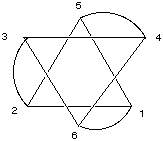Look at the following alternate representation of this molecule to make sure you are visualizing it correctly.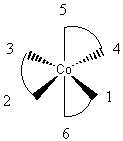A. Does this molecule possess one or more C2 axes of rotation? If so, where are they and how many are there?

B. Does this molecule possess one or more C3 axes of rotation? If so, where are they and how many are there?

C. Does this molecule possess an inversion center?

D. Does this molecule possess one or more reflection planes? If so, where are they and how many are there?

3.* (1997 1 6) Aluminum (III) hexafluoride anion, AlF63-, has an octahedral structure in which the Al3+ cation is located at the center and the six F- anions are located at the mid points of the six faces of a cube:

Fill in the following table with information about the structure of AlF63-. To receive partial credit describe the symmetry elements you have found.

 Symmetry element How many? C2 axes C3 axes C4axes C6axes S3axes S4axes S6axes inversion center?

Is this molecule polar? Chiral?

4.* (1996 1 2) List all the symmetry elements of Ru(bipy)32+ (shown below). What is its point group? Does it have a dipole moment? Is it chiral?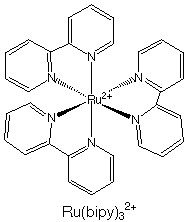5.* (1995 1 4) Are all chiral molecules polar? Are all polar molecules chiral? What are the conditions for a molecule to be nonpolar? What are the conditions for a molecule to be achiral?

6.* (1994 F 1A)

What is the point group of each of the following disubstituted cyclobutanes? Assume that cyclobutane itself has D4h symmetry and that substituting H by Cl changes no other structure parameters. Which structures are polar and which chiral?

A. 1,1-dichlorocyclobutane
B. cis 1,2-dichlorocyclobutane
C. trans 1,2-dichlorocyclobutane
D. trans 1,3-dichlorocyclobutane
E. cis 1,3-dichlorocyclobutane

7. A good rule of thumb is that bonds can only form when both participating orbirtals have the same inversion symmetry about the internuclear axis. A. In what relative positions must an s and p orbital be in order to bond? What is the symmetry of each about the internuclear axis?

B. In what relative positions would s and p orbitals have cancelling overlap?

C. Which of the following orbitals could form bonds with each other?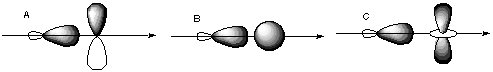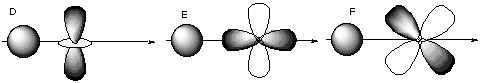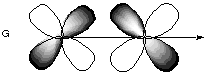8.* (1994 1 1) Shown below are the one s, three p, and five d atomic orbitals with their phases indicated by shading. Pay special attention to the axes, which are not all the same!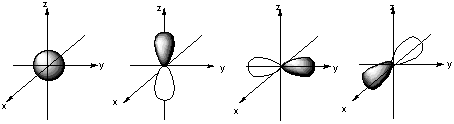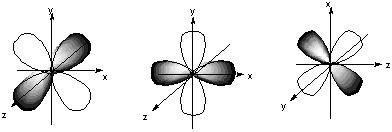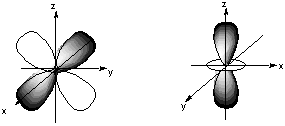A. Classify these nine atomic orbitals by their symmetry under inversion, I, that is, by their change in a center of symmetry. Which of these atomic orbitals are even (do not change sign) and which are odd (do change sign) under inversion?

B. Consider a py atomic orbital on one nuclear center A and one of the five d atomic orbitals on another nuclear center B. Let the nuclear centers A and B be placed on the x axis with A on the left and B on the right. As A and B approach, molecular orbitals can be formed from the two atomic orbitals if these two atomic orbitals have the same symmetry with respect tot he internuclear axis. With which atomic d orbital on B is there a nonzero (noncancelling) overlap with the atomic py orbital on A?

C. Draw pictures of the bonding and anitbonding molecular orbitals that result from this linear combination of atomic orbitals.

D. Classify the resulting molecular orbital as to its symmetry with respect to the internuclear axis.

9.* (1993 1 1) Allene (1,2-propadiene) has the formula C3H4 and consists of three carbon atoms connected by double bonds and two pairs of terminal H atoms: CH2=C=CH2.

A. Draw a picture looking down the C-C-C axis showing the orientation of the two filled pi orbitals.

B. Are the two CH2 planes parallel or perpedicular to one another?

C. Name each symmetry element present for allene. Name each element as many times as different kinds of the same type exist in the molecule, e.g. if there are two different (noncoinciding) C2 rotation axes, name C2 twice. Do not guess; wrong answers will be subtracted from right answers.

D. What is the formal charge on the central carbon atom in allene?

E. Is the allene molecule polar? Explain.

10. If molecular orbitals have similar energies are the same symmetry, they can mix with each other. Which of the following molecular orbitals could mix with each other?

A.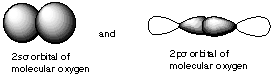B.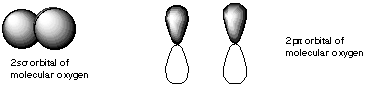C.